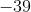# High School Math : Parabolas

## Example Questions

### Example Question #5 : Pre Calculus

Find the vertex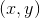for a parabola with equation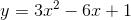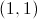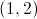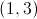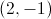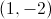Explanation:

For any parabola of the form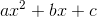,  the-coordinate of its vertex is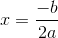So here, we have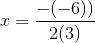We plug this back into the original equation to find: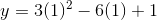### Example Question #6 : Pre Calculus

What is the minimal value of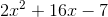over all real numbers?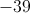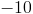No minimum value.Explanation:

Since this is an upwards-opening parabola, its minimum value will occur at the vertex.  The-coordinate for the vertex of any parabola of the form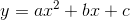is atSo here,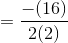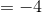We plug this value back into the equation of the parabola, to find the value of the function at this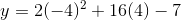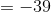Thus the minimal value of the expression is